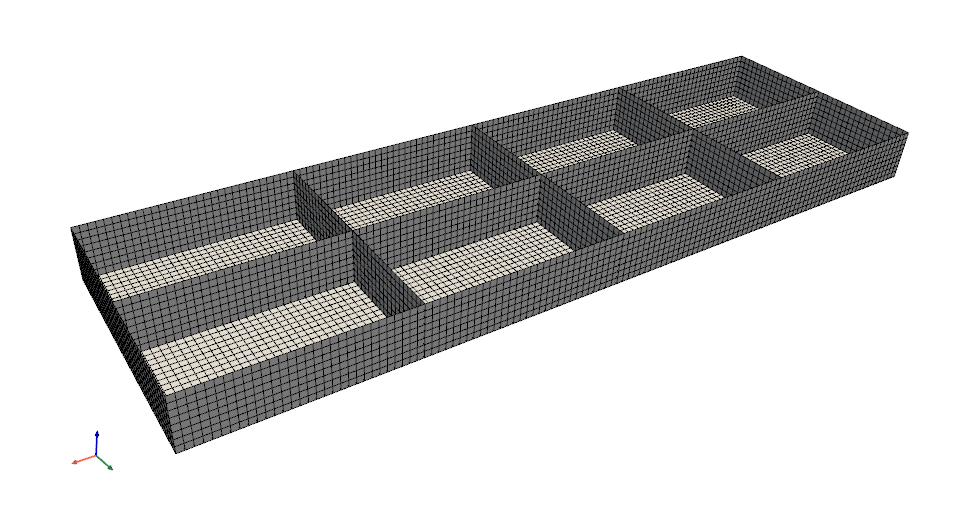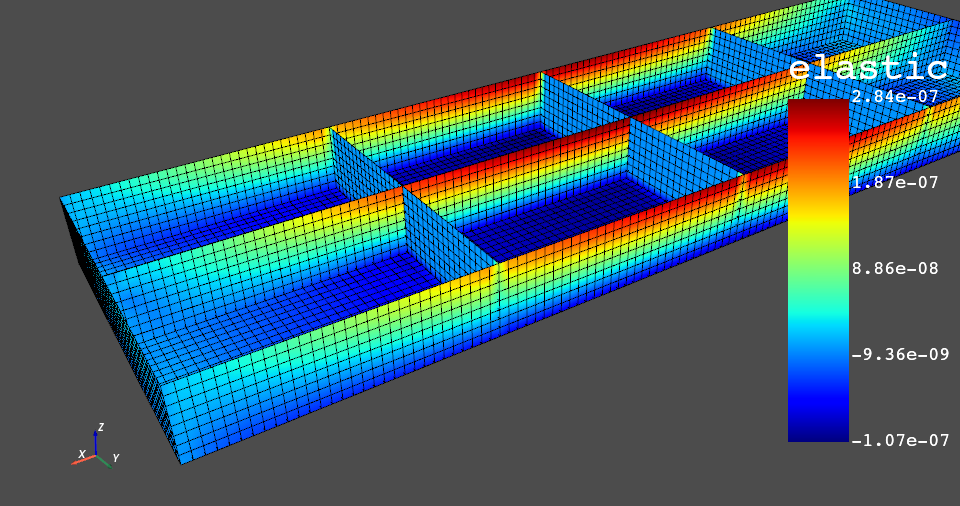# Plot#

DPF-Core has a variety of plotting methods for generating 3D plots of Ansys models directly from Python. These methods use VTK and leverage the PyVista library to simplify plotting. For more information, see the PyVista Documentation.

## Plot the mesh from the `Model` object#

The `Model.plot()` method plots the mesh of the model immediately after loading it.

This example downloads a simple pontoon mesh from the internet and uses the `ansys.dpf.core.model` class to load it:

```>>> import ansys.dpf.core as dpf
>>> from ansys.dpf.core import examples
>>> model = dpf.Model(filename)
>>> model.plot()
```The default plotter settings display the mesh with edges shown and lighting enabled. For a list of all keyword arguments, see plot.

## Plot the meshed region#

The `MeshedRegion.plot()` method plots the meshed region. If the meshed region is generated from the model’s metadata, the plot generated is identical to the plot generated by the `Model.plot()` method.

Plot the meshed region:

```>>> mesh = model.metadata.meshed_region
>>> mesh.plot()
```When a field is provided as the first argument, the mesh is plotted using these values.

This example extracts the nodal strain in the X direction:

```First, extract the X component strain

>>> strain = model.results.elastic_strain()
>>> fields = strain.outputs.fields_container()
>>> field = fields.select_component(0)
>>> print(field)
DPF elastic_strain_1.s0 Field
Location: ElementalNodal
Unit:
8640 entities
Data:1 components and 69120 elementary data

This ElementalNodal strain must be converted to nodal strain for
it to be plotted.

>>> nodal_field = field.to_nodal()
>>> mesh.plot(nodal_field)
```Note

Only fields with `Nodal` and `Elemental` locations are supported. Use the `to_nodal` operator to convert to the `Nodal` location or the `ansys.dpf.core.operators.averaging.nodal_to_elemental` class to convert to the `Elemental` location.